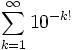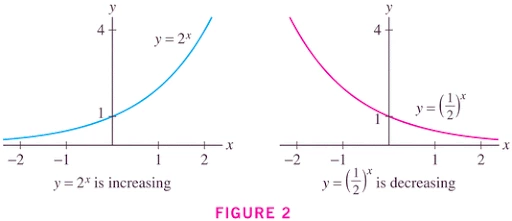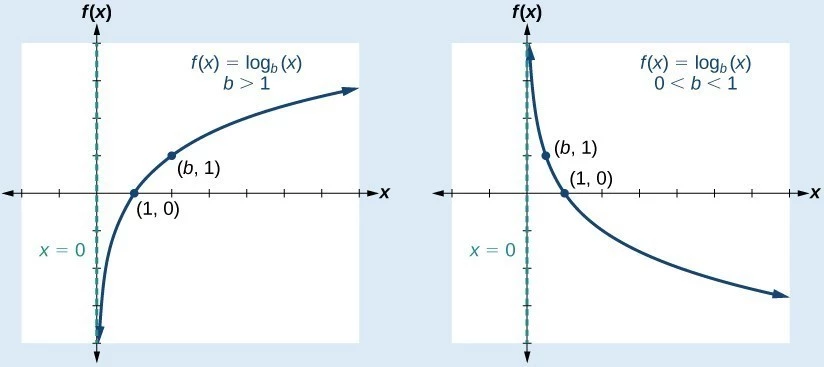Class 11
MATHS
Fundamental Of Mathematics

# Transcendental NumberAnswer

Step by step solution by experts to help you in doubt clearance & scoring excellent marks in exams.

Updated On: 30-9-2020Apne doubts clear karein ab Whatsapp par bhi. Try it now.
CLICK HERELoading DoubtNut Solution for you

Watch 1000+ concepts & tricky questions explained!86.4 K+4.3 K+

Related Videos98743921

800+

16.3 K+

2:371178453

16.0 K+

155.3 K+

2:3751575

6.0 K+

120.4 K+

1:001408586

10.0 K+

201.6 K+

1:043638963

144.1 K+

145.1 K+

3:001527856

182.0 K+

211.6 K+

1:2319910481

7.2 K+

145.2 K+

2:131408589

18.1 K+

363.5 K+

0:4823655

12.2 K+

244.8 K+

5:1123273139

8.1 K+

163.3 K+

2:05

Very Important Questions

Trending Chapters

Jee 2021 | Division And Distributions-Iii | Examples | Important Results Of Straight Lines | Allied Angles | Examples | Learn Different Forms of Equations of Circles in One Class | Introduction To Cartesian Coordinate Geometry | Introduction | Imaginary Number | Powers Of Iota | Complex Numbers | Examples | Jee | Permutation And Combinations | L1 | Factorial Notation | Examples | Definition And Different Forms Of Straight Line | Introduction | Inequalities | Algebraic Solution and Graphical Representation of Linear Inequalities in one Variable | Examples

## Transcendental Function

In this article, we will focus on transcendental function where we will look upon definition of transcendental number and number along with its detailed examples. At the end of the article, a brief concept on algebraic function is stated with the respective substantial examples.

The special case of a relation can be termed as function. Let us understand this statement precisely. Assume X, Y as two non-empty sets with R as a relation from X to Y. Here, R may either relate an element of X to more than one element of Y or it may not relate any element of X to any element of Y. However a function is supposed to relate every element of X to a distinctive element of Y.

A real valued function is the function that has either R or one of its subsets as a range. Thus, the outcome of domain as R or a subset of R gives us real function. In other words, assume R as a set of all real numbers with X, Y as non-empty subsets of R. For every rule ‘f’ that associates to each xX, a distinctive element y of Y is termed as real valued function of the real variable or simply, a real function and we can state this mathematically as-

f: X → Y

The standard real functions are classified into two categories which are further divided into several sub-types:

1. Algebraic Function

1. Polynomial function
2. Rational function
3. Irrational function
4. Modulus function
5. Signum function
6. Greatest integer function
7. Fractional part function

2. Transcendental Function

1. Logarithmic function
2. Exponential function
3. Trigonometric function
4. Inverse trigonometric function

## What is a Transcendental Number?

We know that a real number has two sub types: rational and irrational number. A rational number is the one that can be stated as the division of one whole number by another whole number. In decimal form, the rational number either be terminating or recurring in nature. On the other hand, the irrational number is the one that cannot be stated as the division of one whole number to another and possess a non-recurring decimal form.

The square root 2 and pi are both irrational in nature however pi can be considered in the category of transcendental number but not the square root of 2.  This is because of the root or solution of a polynomial with integer coefficients.

Example: x2 - 2 = 0

x2 = 2

x = +√2 or -√2

Here root 2 or square root of all integers along with the associated rational numbers is algebraic number. On the other hand, pi is not the root of any polynomial with integer coefficients so it comes under the transcendental number category.

The concept of transcendental number was brought up by a Swiss Mathematician Leonhard Euler in 18th century but the existence of this number was proved by a French Mathematician Joseph Liouville in 1844. He did the discovery by naming it after his name Liouville constant.= 0.11000100000000000000000100……

Thus, e was proved as a transcendental number in 1874 whereas π was considered as a transcendental number in 1882.

Π * e and eπ is proved as transcendental number but π-e, π+e and πe are yet unproven.

The discovery of the Gelfond-Schneider theorem was first introduced independently in 1934 by the Soviet mathematician Alexander Gelfond and German mathematician Theodor Schneider. The theorem states that the value of ab will be transcendental if a and b are algebraic numbers (a0 or1) and b is irrational.

Related Videos:

## Transcendental Function Definition and Examples

Transcendental functions are those functions that transcend algebra in the sense that they cannot be built up in the finite number of steps involving elementary operations like addition, multiplication and extraction of roots. Some examples of these functions are:

f(x) = xπ

f(x) = cx

f(x) = xx

f(x) = x√x

f(x) = logcx

f(x) = sin x

Let us explore the types if transcendental functions in detail:

1. Exponential Function

The general exponential function f is defined by f(x) = ax if a is any positive real number with Df (domain of f) = R

Some of the properties of the function f(x) = ax (a>0, xR) are:

1. a0 =1
2. ax . ay = ax+y for all x, y R
3. (ax)y = axy for all x, y R
4. a-x = 1/ax for all x RIn particular, if a = 1 then f(x) = 1x = x, which is a constant function

One should know that if a ≠ 1, then Rf (range of f) = (0, ∞) and if a =1, then Rf = {1}

Thus, the function f(x) = ex is called natural exponential function if a = e i.e. the base is e (an irrational number whose approximate value is 2.71828)

Thus, Df (domain of f) = R and Rf (range of f) = (0, ∞)

2. Logarithmic Function

The logarithmic function with base ‘a’ is denoted by the symbol logax if a is a positive real number, a ≠ 1 and x is a non-negative number.

Mathematically, y = logax and only if x = ay

Thus, the domain of logarithmic function is (0, ∞) and range is R on the basis of below graph.Thus, the function f(x) = logex is labelled as natural logarithmic function if the base is e. The function can be denoted by log x or ln x.

Some of the properties of the function logax (a>0, ≠1) are:

1. Domain is (0, ∞) and Range is R
2. loga1 = 0 and logaa =1
3. logaxy = logax +logay, x>0 and y>0

## Algebraic Function

The root of any polynomial with an integer coefficient is the algebraic function. In other words, it is the reverse mechanism of transcendental function.

The detailed explanation of the examples of this function is:

1. Polynomial function

A function can be defined as polynomial function if f is defined by f(x) = a0 + a1x + a2x2 + ….. + anxn. In this case, a1,a2, …. an are real numbers and n is a positive integer.

A linear function is the one with n= 1 and if n=2, it is termed as quadratic function. Let us understand this through an example:

f(x) = 4 - 9x is a linear function whereas the example of quadratic function is f(x) = 5 + √5x – 2x2.

2. Rational Function

A rational function is the function that can be represented as the quotient of two polynomial function, i.e. f(x) = g(x)/h(x) where g(x) and h(x) are the polynomial functions and h(x) ≠ zero.

Important Chapters:

By using this site you agree to the use of cookies. Check our Cookie Policy for more details